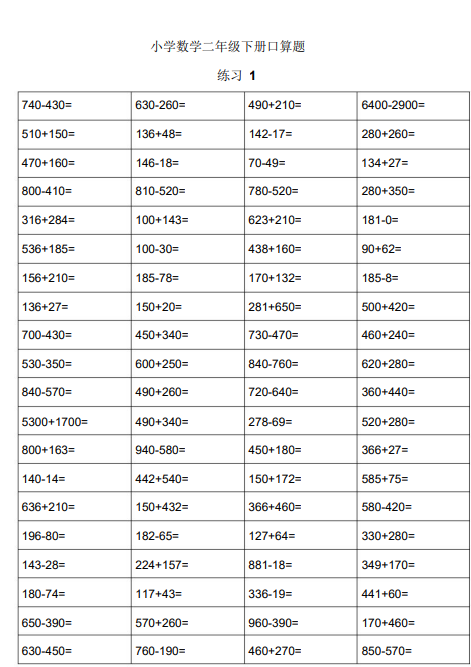740-430= 630-260= 490+210= 6400-2900=
510+150= 136+48= 142-17= 280+260=
470+160= 146-18= 70-49= 134+27=
800-410= 810-520= 780-520= 280+350=